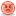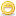# Thread: ROW SIZE / Column Size

1. Hi,

There are lot of threads in Metalink about how much bytes Oracle really calculate while calcultaing actual Row Length.

Does someone have exact and realistic information about how many extra length bytes oracle considers for the following data types.

CHAR, VARCHAR2, DATE, NUMBER, RAW, ROWID, LONG, BLOB, CLOB.

Below given is an example table.

Table A has foll. columns.

colA char(3)
colB varchar2(3)
colC number(5,2)
colD date
colE raw(25)
colF rowid
colG long
colH blob
colI clob

How much byte size Oracle considers against each column to calculate RowSize?

This would help lot of users in sizing objects.

TIAReply With Quote

2. Calculating exact rowsize.
I was reading one of the docs on metalink regarding this and phew.. it isn't that simple..
Posting the excerpts from Metalink...

----
Step 1: Calculate the Total Block Header Size
The space required by the data block header is the result of the following formula:

Space after headers (hsize) = db_block_size - kcbh - ub4 - ktbbh - ((INITRANS - 1) * ktbit) - kdbh

Where: DB_BLOCK_ SIZE is the database block size as viewed in the V\$PARAMETER view, kcbh, ub4, ktbbh, ktbit,kdbh are constants whose sizes you can obtain by selecting from entries in the V\$TYPE_SIZE view ,INITRANS is the initial number of transaction entries allocated to the table

Step 2: Calculate the Available Data Space Per Data Block
The space reserved in each data block for data, as specified by PCTFREE, is calculated as follows:

available data space (availspace) = CEIL(hsize * (1 - PCTFREE/100)) - KDBT

Where: CEIL rounds a fractional result to the next highest integer
PCTFREE is the percentage of space reserved for updates in the table
KDBT is a constant whose size you can obtain by selecting the entry from the V\$TYPE_SIZE view

Note: If you are unable to locate the value of KDBT, use the value of UB4 instead.

Step 3: Calculate the Space Used per Row

Calculating the amount of space used per row is a multi-step task.

First, you must calculate the column size, including byte lengths:

Column size including byte length = column size + (1, if column size < 250, else 3)

Note: You can also determine column size empirically, by selecting avg(vsize(colname)) for each column in the table.

Then, calculate the row size:

Rowsize = row header (3 * UB1) + sum of column sizes including length bytes

Finally, you can calculate the space used per row:

Space used per row (rowspace) = MAX(UB1 * 3 + UB4 + SB2, rowsize) + SB2

Where:
UB1, UB4, SB2 are constants whose size can be obtained by selecting entries from the V\$TYPE_SIZE view

When the space per row exceeds the available space per data block, but is less than the available space per data block without any space reserved for updates (for example, available space with PCTFREE=0), each row will be stored in its own block.

When the space per row exceeds the available space per data block without any space reserved for updates, rows inserted into the table will be chained into 2 or more pieces, hence, this storage overhead will be higher.

Step 4: Calculate the Total Number of Rows That Will Fit in a Data Block

You can calculate the total number of rows that will fit into a data block using the following equation:

Number of rows in block = FLOOR(availspace / rowspace)

Where:
FLOOR rounds a fractional result to the next lowest integer

In summary, remember that this procedure provides a reasonable estimate of a table's size, not an exact number of blocks or bytes. After you have estimated the size of a table, you can use this information when specifying the INITIAL storage parameter (size of the table's initial extent) in your corresponding CREATE TABLE statement.

Space Requirements for Tables in Use

After a table is created and in use, the space required by the table is usually higher than the estimate derived from your calculations. More space is required due to the method by which Oracle manages free space in the database
----

SanjayReply With Quote

3. ##[QUOTE][i]First, you must calculate the column size, including byte lengths:

Column size including byte length = column size + (1, if column size < 250, else 3)

Hi Sanjay,

Still my question is How much byte size Oracle considers against each column below for its data type to calculate RowSize?

colA char(3)
colB varchar2(3)
colC number(5,2)
colD date
colE raw(25)
colF rowid
colG long
colH blob
colI clob

I don't think that foruma "Column size including byte length = column size + (1, if column size < 250, else 3)" is same for all the data types.

Any more inputs?

Thanks any way.Reply With Quote

4. Hi all,

Pl. don't let this thread die...

It really requests brain storming thought from all of you Gurus.

Thanks,Reply With Quote

5. ##Hi guys,

At last, I myself have found the answer to my thread with the help of Oracle/Metalink.

Here is the reply.
--------------------------------------------
The space usage in a table row will depend on the data inserted into that row.For your columns the size will be as follows:

colA char(3) - will occupy 3 bytes.
colB varchar2(3) - will occupy 1 byte for each character inserted.
colC number(5,2) - Each digit takes up half a byte. One byte is used for the exponent and another byte is appended if the number is -ve.So here it would take 5 bytes - 3 bytes for the digits, one byte for the exponent and one extra byte if the number is negative.
(Note: 1031902.6 How Does Oracle Store Internal Numeric Data? )
colD date - this is a fixed length datatype that always takes 7 bytes.
(Note: 69028.1 How does Oracle store the DATE datatype internally? )
colE raw(25) - depends on the data inserted.The size can be found using utl_raw.length (Note:77327.1 - Using UTL_RAW Package).
colF rowid - will occupy 10 bytes after Oracle 8.The only time you have to add space for the rowid, is if you create a column of type rowid. The space taken by the rowid value that Oracle generates is not added in when estimating the size a table needs.
colG long, colH blob, colI clob - again depends on the data inserted.
Note:94194.1 - HOW TO CALCULATE LENGTH OF LONG COLUMN
Note:61737.1 - How to Manipulate Large Objects Using DBMS_LOB Package
Using these you can find the sizes of these datatypes.

Apart from these a column whose size is less than 250, would use 1 length byte, otherwise it would use 3 bytes - this applies
to both variable and fixed length columns.If a column contains a NULL value and it is not the trailing column (last one defined in a table), it will use 1 byte. If it is the trailing column, then no space is used by that column.

Considering all these the SQL statement to calculate the average row size of rows in a table that already exists would be:

Select (avg(vsize(nvl(column1,0))) + 1) + (avg(vsize(nvl(column2,0))) + 1) + ...(avg(vsize(nvl(columnN,0))) + 1) + 3 from table_name;

You can also find the same from avg_row_length column of user_tables after analysing the table.

And finally to know the actual size occupied by the various column types of a table you can dump the datablock containing some rows and read the dump file from the user trace and find out the size information by associating it with the column no and type.Anyway for interpreting the column value you'll need to convert it from hexadecimal to readable format.

Hope you guys/gurus get help and good knowledge from this thread.

Anyway Thanks,Reply With Quote

####Posting Permissions

• You may not post new threads
• You may not post replies
• You may not post attachments
• You may not edit your posts
•

Click Here to Expand Forum to Full Width